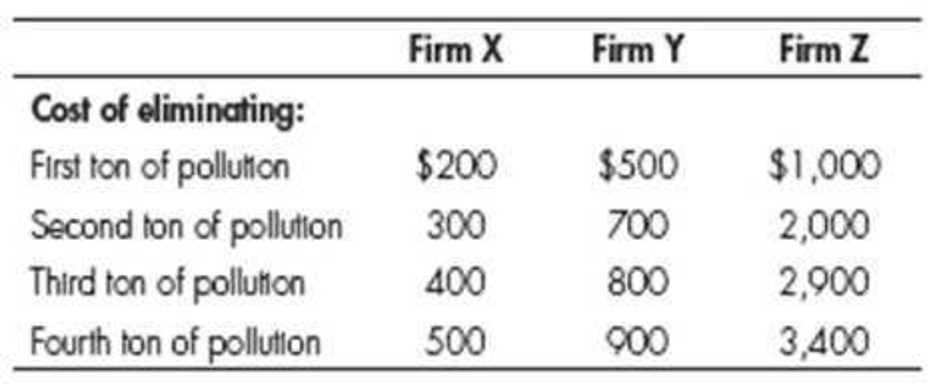Chapter 30, Problem 3WNG

Chapter
Section
Textbook Problem

Using the following data, prove that pollution permits that can be bought and sold can reduce pollution from 12 tons to 6 tons at a lower cost than a regulation which specifies that each of the three firms must cut its pollution in half.To determine

Prove that, by selling and buying pollution permit can reduce half ton pollution.

Explanation

Table 1 shows the cost of eliminating pollution for Firm X, Y and X.

 Firm X Firm Y Firm Z First ton of pollution $200$500 \$1,000 Second ton of pollution 300 700 2,000 Third ton of pollution 400 800 2,900 Fourth ton of pollution 500 900 3,400

The maximum cost decrease due to eliminating third and fourth ton of pollution is calculated as follows.

Cost=((Third ton of pollution+Fourth ton of pollutionFirm A)+(Third ton of pollution+Fourth ton of pollutionFirm B)+(Third ton of pollution+Fourth ton of pollutionFirm C))=

Still sussing out bartleby?

Check out a sample textbook solution.

See a sample solution

The Solution to Your Study Problems

Bartleby provides explanations to thousands of textbook problems written by our experts, many with advanced degrees!

Get Started

Find more solutions based on key concepts